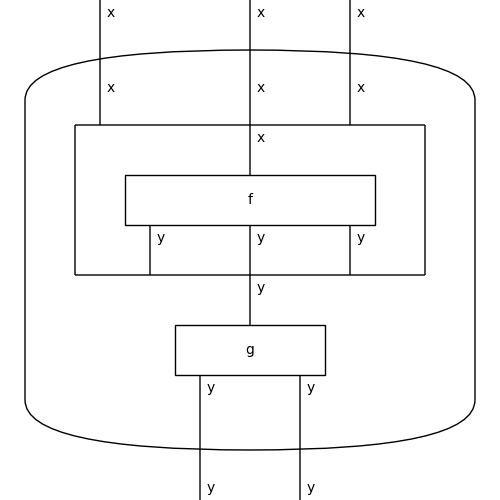# Bubble#

class discopy.monoidal.Bubble(arg, dom=None, cod=None, **params)[source]#

A bubble is a box with a diagram `arg` inside and an optional pair of types `dom` and `cod`.

Parameters:
• arg (Diagram) – The diagram inside the bubble.

• dom (T) – The domain of the bubble, default is that of `other`.

• cod (T) – The codomain of the bubble, default is that of `other`.

Examples

```>>> x, y = Ty('x'), Ty('y')
>>> f, g = Box('f', x, y ** 3), Box('g', y, y @ y)
>>> d = (f.bubble(dom=x @ x, cod=y) >> g).bubble()
>>> d.draw(path='docs/_static/monoidal/bubble-example.png')
```